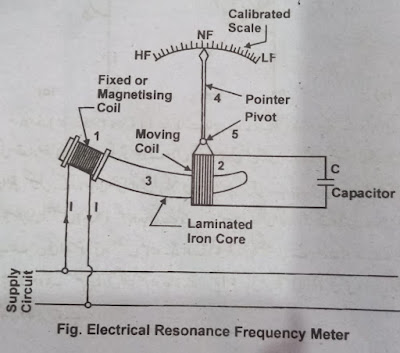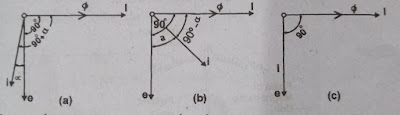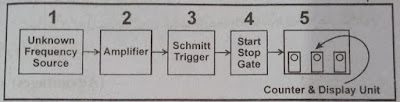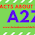# Frequency Meters-Frequency Meter Types and Construction-Digital Frequency Meter-Special Instruments

This article provides detailed information about Frequency Meters and Types of Frequency Meters, Electrical Resonance Frequency Meter, Mechanical Resonance Frequency Meter, Weston Frequency Meter, and Digital Frequency Meter. Also, the structure, working principle, working method, and benefits of all these instruments are explained.

# Frequency Meters

Frequency meters are meters that are used to directly measure the frequency of power circuits. The meters or instruments used are called frequency meters. Such frequency meters are designed to measure the frequency of electronic circuits. Which can measure very high frequencies. Because the frequency range in electronics circuits can be up to several megahertz. Electrical circuits have a very low-frequency range (25c/s to 60c/s). Each country's power system has its standard frequency. The standard frequency s 50c / s / 600 is being used in most countries. The standard frequency of the power system in Pakistan is 50c/s±1%.

## Types of Frequency Meters

• Electrical Resonance Frequency Meters
• Mechanical Resonance Frequency Meters
• West or Moving Iron Frequency Meters
• Ratio Type Frequency Meters
• Digital Frequency Meters

## Electrical Resonance Frequency Meters

### Working Principle

Electrical resonance frequency meter, frequency meter works based on the principle of electrical resonance. Teaching frequency in an AC circuit increases the inductive activity "XL". While the capacitor activity "XC" is low. The special frequency at which the XL and XC of the circuit are equal is called electrical resonance. And the frequency at which the resonance occurs is called the frequency correlation frequency. The movement of the moving coil in the electrical resonance frequency meter changes its XL.

### Construction

The structure of this instrument is shown in the figure below. This instrument consists of a fixed or magnetizing coil and a moving coil. The magnetizing coil is winded with a single wire bar at one end of a curved laminated core. This coil is connected parallel to this supply. The frequency of which has to be measured. The second moving coil is pivoted near the core of the fixed coil in such a way that when the torque acts on it, it can be near or far from the fixed coil. A pointer with a peanut coil is also a fix. Indicates the relative value of the frequency by moving on the scale calibrated in the frequency. Unlike moving coil power factor meters, it also does not have control torque. There is also a capacitor of appropriate value parallel to the moving coil.Frequency Meter

### Working Method

This meter contains different quantities of the value supply frequency of the moving coil castor action (CX). Because the moving coil is not directly connected to the supply. But its inductance varies depending on the movement of the value moving coil of inductive reactance (XL). As the moving coil gets closer to the fixed coil its inductance activity increases and when the moving coil moves away from the fixed coil (magnetic tying coil) its value of XL decreases. When the fixed coil is connected to the supply, a current I starts running in it. Due to this current, a flux ɸ runs in the iron core. Which is in the phase of the current. Due to this flux, a voltage (e) is induced in the moving coil. These voltage fluxes are leaking from ɸ to 90.

The current flowing due to voltage (e) in the moving coil can also lead to its voltage α angle. And this α angle can also be leading. As shown in Figures a and b. Angle α Refers to the phase angle between the voltage (e) and current generated in the moving coil. The value of angle α depends on whether the inductance of the moving coil is high or the capacitance is high. (Because the inductance of the moving coil may vary depending on its position.) The torque coming on the coil (90± α) is directly proportional to i.Cos, i.e. the torque depends on the angle α.Angle Working Method

• This instrument can be made very sensitive to different amounts of frequency.
• They are simple in structure.
• Don't use too much power.

## Mechanical Resonance Frequency Meters

Mechanical resonance frequency meters are also called vibration radio frequency meters. Because the leaves in it show the corresponding frequency by vibrating.

### Working Principle

The working principle of the mechanical resonance frequency meter is based on the mechanical resonates of the fine particles. And all the leaves try to tremble. But most of them are vibrations that have a natural mechanical frequency that matches the frequency of the magnetic field.

### Construction

These instruments are very simple in structure. They contain an electromagnet. It is made by winding a steel bar on a single laminated iron core and winding a copper bar with a single wire. A resistor is attached to the series and it is connected to the supply sides (Across). And the frequency is determined. In this sense, the external connections of this meter are like a voltmeter. Numerous steel sheets or reeds on one side of the core are fixed in a line along with a base plate. These metal sheets are about 4 mm wide and 0.5 mm thick. These leaves are not the same but their length and width are slightly different. They have weights or flags of different values ​​on their upper ends.Construction/Parts

## Digital Frequency Meters

The digital frequency meter counts the number of supply cycles per second and displays them directly on the screen in decimal numbers.

Like other digital instruments, digital frequency meters have many advantages over analog frequency meters (mentioned earlier). (Details can be found in the Digital Instruments chapter). For example, they are free from parallax error, zero adjustments, and the position of use. They are more sensitive, more accurate, and have higher resolution. In addition, they are light in weight and smart, use less power, and set their polarity and range. Digital signals can be further processed.

### Explanation & Working Principle

The figure shows the block diagram of a digital frequency meter. The basic working method of this meter is to determine the frequency of the supply source. The units of this supply are converted into Train of Pulses through a special type of trigger circuit. A pulse is formed from one cycle of supply. In this way, the supply sine wave cycles are converted to square wave pulses in the shape shown on Sine (Wave Cycles). Therefore these square wave pulses are considered as alternatives to the supply cycle. These pulses appearing are counted by an electronic counter, and the supply frequency is displayed directly on the screen in decimal geometric form. In the block diagram shown, the source indicates the frequency to be determined.Digital Frequency Meter Diagram

1.1.2.1.